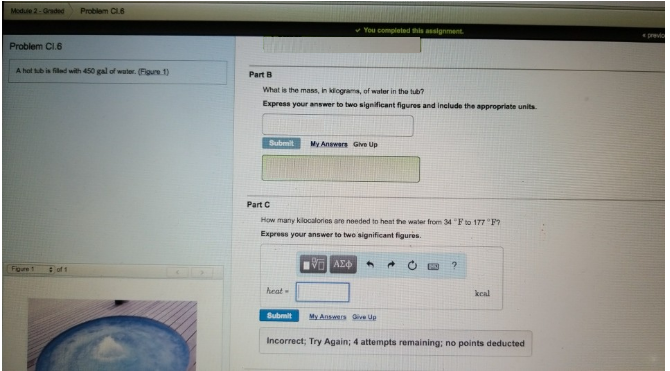# Problem: A hot tub is filled with 450 gal of water. (Figure 1) Part B What is the mass, in kilograms, of water in the tub? Express your answer to two significant figures and include the appropriate units. Part C How many kilocalories are needed to heat the water from 34°F to 177°F? Express your answer to two significant figures.

###### FREE Expert Solution
86% (481 ratings)###### Problem Details

A hot tub is filled with 450 gal of water. (Figure 1)

Part B

What is the mass, in kilograms, of water in the tub?

Express your answer to two significant figures and include the appropriate units.

Part C

How many kilocalories are needed to heat the water from 34°F to 177°F?